# GED Math : Supplementary Angles

## Example Questions

### Example Question #521 : 2 Dimensional GeometryIn degrees, what is the measure of the smallest angle in the figure above?Explanation:Start by solving for. Since all three angles lie on a straight line, the three angles must add up to. We can then write the following equation to solve for: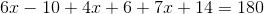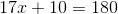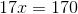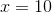Now, plug in the values ofto find the angle measurements.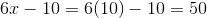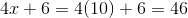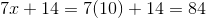The smallest angle isdegrees.

### Example Question #41 : Supplementary Angles

Which of the following angles forms a supplementary angle pair with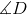?

Given that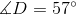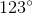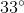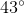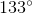Explanation:

Which of the following angles forms a supplementary angle pair with?

Given thatSupplementary angles sum up to 180 degrees. Therefore, to find our answer, we simply need to do the following: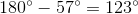So, our answer is### Example Question #521 : Geometry And Graphs

What is the measure of an angle supplementary to an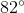angle?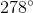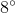No solution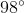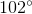Explanation:

First we need to know that supplementary angles, when added, equal toSo when we have the degree measurement of one angle, all we need to do is subtract it from the total (which is 180) and that will give us the measurement of our supplementary angle.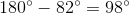### Example Question #42 : Supplementary Angles

In degrees, find the measure of the smallest angle in the figure below.Explanation:Recall that when angles are supplementary, the will add up to. Thus, we can write the following equation: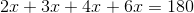Solve for.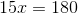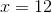Now, the smallest angle in the figure is,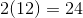.

The smallest angle must be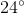.

### Example Question #45 : Supplementary Angles

Find the measure of angle B if it is the supplement to angle A: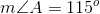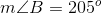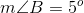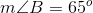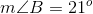Explanation:

If two angles are supplementary, that means the sum of their degrees of measure will add up to 180. In order to find the measure of angle B, subtract angle A from 180 like shown: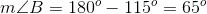This gives us a final answer of 65 degrees for angle B.

### Example Question #46 : Supplementary Angles

Find the measure of angle B if it is the supplement to angle A: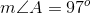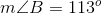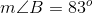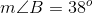Explanation:

If two angles are supplementary, that means the sum of their degrees of measure will add up to 180. In order to find the measure of angle B, subtract angle A from 180 like shown: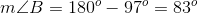This gives us a final answer of 83 degrees for angle B.

### Example Question #47 : Supplementary Angles

Find the measure of angle B if it is the supplement to angle A: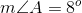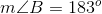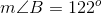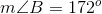Explanation:

If two angles are supplementary, that means the sum of their degrees of measure will add up to 180. In order to find the measure of angle B, subtract angle A from 180 like shown: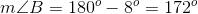This gives us a final answer of 172 degrees for angle B.

### Example Question #48 : Supplementary Angles

Find the measure of angle B if it is the supplement to angle A: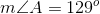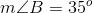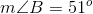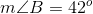Explanation:

If two angles are supplementary, that means the sum of their degrees of measure will add up to 180. In order to find the measure of angle B, subtract angle A from 180 like shown: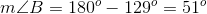This gives us a final answer of 51 degrees for angle B.

### Example Question #49 : Supplementary Angles

Find the measure of angle B if it is the supplement to angle A: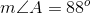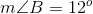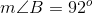Explanation:

If two angles are supplementary, that means the sum of their degrees of measure will add up to 180. In order to find the measure of angle B, subtract angle A from 180 like shown: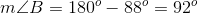This gives us a final answer of 92 degrees for angle B.

### Example Question #50 : Supplementary Angles

Find the measure of angle B if it is the supplement to angle A: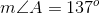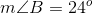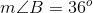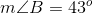Explanation:

If two angles are supplementary, that means the sum of their degrees of measure will add up to 180. In order to find the measure of angle B, subtract angle A from 180 like shown: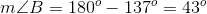This gives us a final answer of 43 degrees for angle B.

### All GED Math Resources